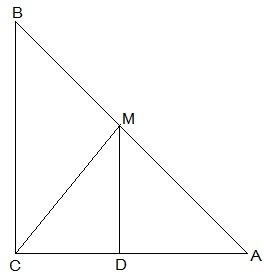Guru

# ABC is a triangle right angled at C. A line through the mid-point M of hypotenuse AB and parallel to BC intersects AC at D. Show that (i) D is the mid-point of AC (ii) MD ⊥ AC Q.7(1-2)

• 0

Yesterday i was doing the question from class 9th ncert book of math of Quadrilaterals chapter of exercise 8.2   What is the easiest way for solving it because i was not able to do this question please help me for solving this question ABC is a triangle right angled at C. A line through the mid-point M of hypotenuse AB and parallel to BC intersects AC at D. Show that (i) D is the mid-point of AC (ii) MD ⊥ AC

Share

1.(i) In ΔACB,

M is the midpoint of AB and MD || BC

, D is the midpoint of AC (Converse of mid point theorem)

(ii) ∠ACB = ∠ADM (Corresponding angles)

also, ∠ACB = 90°

, ∠ADM = 90° and MD ⊥ AC

• 0